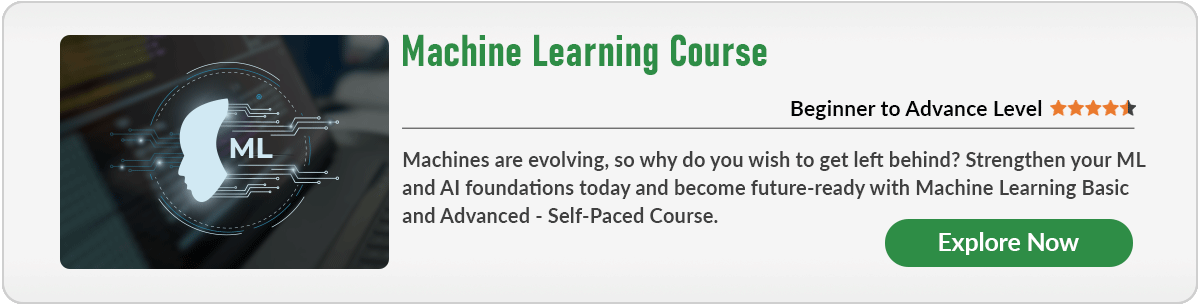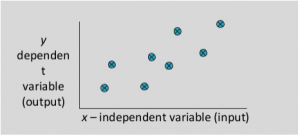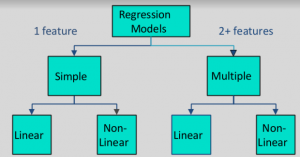# Regression and Classification | Supervised Machine Learning

• Difficulty Level : Hard
• Last Updated : 18 May, 2022

What is Regression and Classification in Machine Learning?

Data scientists use many different kinds of machine learning algorithms to discover patterns in big data that lead to actionable insights. At a high level, these different algorithms can be classified into two groups based on the way they “learn” about data to make predictions: supervised and unsupervised learning.Supervised Machine Learning: The majority of practical machine learning uses supervised learning. Supervised learning is where you have input variables (x) and an output variable (Y) and you use an algorithm to learn the mapping function from the input to the output Y = f(X) . The goal is to approximate the mapping function so well that when you have new input data (x) that you can predict the output variables (Y) for that data.
Techniques of Supervised Machine Learning algorithms include linear and logistic regression, multi-class classification, Decision Trees and support vector machines. Supervised learning requires that the data used to train the algorithm is already labelled with correct answers. For example, a classification algorithm will learn to identify animals after being trained on a dataset of images that are properly labelled with the species of the animal and some identifying characteristics.
Supervised learning problems can be further grouped into Regression and Classification problems. Both problems have a goal of the construction of a succinct model that can predict the value of the dependent attribute from the attribute variables. The difference between the two tasks is the fact that the dependent attribute is numerical for regression and categorical for classification.

Regression

A regression problem is when the output variable is a real or continuous value, such as “salary” or “weight”. Many different models can be used, the simplest is the linear regression. It tries to fit data with the best hyper-plane which goes through the points.Types of Regression Models:For Examples:
Which of the following is a regression task?

• Predicting age of a person
• Predicting nationality of a person
• Predicting whether stock price of a company will increase tomorrow
• Predicting whether a document is related to sighting of UFOs?

Solution : Predicting age of a person (because it is a real value, predicting nationality is categorical, whether stock price will increase is discrete-yes/no answer, predicting whether a document is related to UFO is again discrete- a yes/no answer).
Let’s take an example of linear regression. We have a Housing data set and we want to predict the price of the house. Following is the python code for it.

## Python3

 `# Python code to illustrate ``# regression using data set``import` `matplotlib``matplotlib.use(``'GTKAgg'``)``  ` `import` `matplotlib.pyplot as plt``import` `numpy as np``from` `sklearn ``import` `datasets, linear_model``import` `pandas as pd``  ` `# Load CSV and columns``df ``=` `pd.read_csv(``"Housing.csv"``)``  ` `Y ``=` `df[``'price'``]``X ``=` `df[``'lotsize'``]``  ` `X``=``X.values.reshape(``len``(X),``1``)``Y``=``Y.values.reshape(``len``(Y),``1``)``  ` `# Split the data into training/testing sets``X_train ``=` `X[:``-``250``]``X_test ``=` `X[``-``250``:]``  ` `# Split the targets into training/testing sets``Y_train ``=` `Y[:``-``250``]``Y_test ``=` `Y[``-``250``:]``  ` `# Plot outputs``plt.scatter(X_test, Y_test,  color``=``'black'``)``plt.title(``'Test Data'``)``plt.xlabel(``'Size'``)``plt.ylabel(``'Price'``)``plt.xticks(())``plt.yticks(())``  ` ` ` `# Create linear regression object``regr ``=` `linear_model.LinearRegression()``  ` `# Train the model using the training sets``regr.fit(X_train, Y_train)``  ` `# Plot outputs``plt.plot(X_test, regr.predict(X_test), color``=``'red'``,linewidth``=``3``)``plt.show()`

The output of the above code will be:

[Image_Link]https://media.geeksforgeeks.org/wp-content/up

Here in this graph, we plot the test data. The red line indicates the best fit line for predicting the price. To make an individual prediction using the linear regression model:

`print( str(round(regr.predict(5000))) )`

Classification

A classification problem is when the output variable is a category, such as “red” or “blue” or “disease” and “no disease”. A classification model attempts to draw some conclusion from observed values. Given one or more inputs a classification model will try to predict the value of one or more outcomes.
For example, when filtering emails “spam” or “not spam”, when looking at transaction data, “fraudulent”, or “authorized”. In short Classification either predicts categorical class labels or classifies data (construct a model) based on the training set and the values (class labels) in classifying attributes and uses it in classifying new data. There are a number of classification models. Classification models include logistic regression, decision tree, random forest, gradient-boosted tree, multilayer perceptron, one-vs-rest, and Naive Bayes.
For example :
Which of the following is/are classification problem(s)?

• Predicting the gender of a person by his/her handwriting style
• Predicting house price based on area
• Predicting whether monsoon will be normal next year
• Predict the number of copies a music album will be sold next month

Solution : Predicting the gender of a person Predicting whether monsoon will be normal next year. The other two are regression.
As we discussed classification with some examples. Now there is an example of classification in which we are performing classification on the iris dataset using RandomForestClassifier in python. You can download the dataset from Here
Dataset Description

```Title: Iris Plants Database
Attribute Information:
1. sepal length in cm
2. sepal width in cm
3. petal length in cm
4. petal width in cm
5. class:
-- Iris Setosa
-- Iris Versicolour
-- Iris Virginica
Missing Attribute Values: None
Class Distribution: 33.3% for each of 3 classes```

## Python3

 `# Python code to illustrate ``# classification using data set``#Importing the required library``import` `pandas as pd``from` `sklearn.model_selection ``import` `train_test_split``from` `sklearn.ensemble ``import` `RandomForestClassifier``from` `sklearn.preprocessing ``import` `LabelEncoder``from` `sklearn.metrics ``import` `confusion_matrix``from` `sklearn.metrics ``import` `accuracy_score``from` `sklearn.metrics ``import` `classification_report`` ` `#Importing the dataset``dataset ``=` `pd.read_csv(``          ``'https://archive.ics.uci.edu/ml/machine-learning-'``+``          ``'databases/iris/iris.data'``,sep``=` `','``, header``=` `None``)``data ``=` `dataset.iloc[:, :]`` ` `#checking for null values``print``(``"Sum of NULL values in each column. "``)``print``(data.isnull().``sum``())`` ` `#separating the predicting column from the whole dataset``X ``=` `data.iloc[:, :``-``1``].values``y ``=` `dataset.iloc[:, ``4``].values`` ` `#Encoding the predicting variable``labelencoder_y ``=` `LabelEncoder()``y ``=` `labelencoder_y.fit_transform(y)`` ` `#Splitting the data into test and train dataset``X_train, X_test, y_train, y_test ``=` `train_test_split(``              ``X, y, test_size ``=` `0.3``, random_state ``=` `0``)`` ` `#Using the random forest classifier for the prediction``classifier``=``RandomForestClassifier()``classifier``=``classifier.fit(X_train,y_train)``predicted``=``classifier.predict(X_test)`` ` `#printing the results``print` `(``'Confusion Matrix :'``)``print``(confusion_matrix(y_test, predicted))``print` `(``'Accuracy Score :'``,accuracy_score(y_test, predicted))``print` `(``'Report : '``)``print` `(classification_report(y_test, predicted))`

Output:

```Sum of NULL values in each column.
0    0
1    0
2    0
3    0
4    0

Confusion Matrix :
[[16  0  0]
[ 0 17  1]
[ 0  0 11]]

Accuracy Score : 97.7

Report :
precision    recall  f1-score   support
0       1.00      1.00      1.00        16
1       1.00      0.94      0.97        18
2       0.92      1.00      0.96        11
avg/total    0.98      0.98      0.98        45```

References:

My Personal Notes arrow_drop_up